Electricity & Megnetism

# Electrodynamics

Electrodynamics is the branch of Physics that studies the movement of electric charges . The movement of these charges is obtained when an electric potential difference is applied between two points of a conducting medium . The resistance of the medium is the physical property that quantifies the ease with which an electric current is conducted within it. Furthermore, electric current is one of the fundamental quantities of electrodynamics.

Electrodynamics is the field of physics that studies the movement of electric charges.

### → Conductors and insulators

There are materials that are able to conduct electrical charges with relative ease. These materials are called conductors. On the other hand, there are materials that oppose the conduction of electric current, they are called insulators or dielectrics . To learn more, read: Conductors and Insulators .

### → Electric current

The electric current , which is the movement of charges, is calculated by the amount of charges that cross the cross section of a conductor every second. The unit of measurement for electric current in the International System (SI) is the ampere. Its formula is as follows: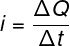i – electric current (A)

ΔQ – electrical charge (C)

Δt – time interval (s)

In conducting materials, electric current is produced by the movement of electrons. These particles are endowed with a negative electrical charge of magnitude 1.6 x 10 -19 C , known as the fundamental electrical charge . Since the electric current is made up of electrons, it can be written as follows: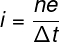n – number of electrons

and – fundamental electrical charge (1.6.10 -19 C)

### → Electrical resistance

The intensity of the electric current formed depends on a characteristic of the body called electrical resistance . Bodies whose electrical resistance is constant, regardless of what voltage is applied to them, are called ohmic. These bodies obey Ohm’s 1st law , described by the following formula:U – electrical voltage (V)

R – electrical resistance (Ω)

Electrical resistance depends on geometric factors such as the length and cross- sectional area of ​​the conductor. This is explained mathematically through Ohm’s 2nd law :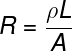L – body length (m)

A – cross-sectional area (m²)

ρ – resistivity (Ω.m)

### → Resistivity

Resistivity, denoted by the symbol ρ , is a microscopic property of the material. The greater the resistivity , the greater the electrical resistance of the body. Thus, conductive materials have low resistivity.

The formula used to calculate resistivity involves microscopic quantities and advanced knowledge of electromagnetic theory, therefore, in high school, we do not usually calculate it, but only use its module, when informed by the exercises.

### → Joule Effect

When electric current flows through an insulating material, we say that a dielectric breakdown has occurred . When an insulating material starts to conduct electricity, a large amount of heat is produced, due to the difficulty that the electric current encounters when passing between the atoms of the material. This heat production phenomenon , known as the Joule Effect , is widely exploited in heaters, showers, electric cookers , etc. The Joule Effect formula allows us to calculate the amount of electrical energy that is dissipated in thermal energy. To do so, we relate quantities such as resistance, electric current and time interval. Watch: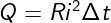Q – amount of energy transformed into heat (J)

R – electrical resistance

### → Electric power

If we want to calculate the amount of energy converted into heat due to the Joule effect , it is necessary to determine the electrical power dissipated. Power is a quantity that measures the rate of energy transfer. Its unit of measurement is the watt , which is equivalent to joule per second.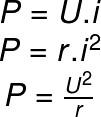P – electrical power (W)

r – electrical resistance (Ω)

## Electrodynamics in Enem

Electrodynamics is the second most popular subject among the Enem questions , so when preparing for the exam, pay special attention to topics such as:

• Ohm’s laws – 1st Ohm’s law and 2nd Ohm’s law ;
• association of resistors ;
• electrical generators ;
• electrical power .

In addition, try to understand the theory behind the content well, as it is as important for the test as the exercise itself. Let’s check an Enem question that involved this content?

(Enem) Every car has a fuse box, which are used to protect electrical circuits. Fuses are made of a material with a low melting point, such as tin, for example, and they melt when an electric current is passed through them equal to or greater than what they are capable of withstanding. The table below shows a series of fuses and the current values ​​supported by them.

 Fuse Electric current (A) Blue 1.5 Yellow 2.5 Orange 5.0 Black 7.5 Red 10.0

A headlight uses a 55 W halogen gas lamp that operates at 36 V. The two headlights are turned on separately, with a fuse for each, but after a malfunction, the driver switched them in parallel, using only one fuse. Thus, assuming that the wiring supports the load of the two headlights, the lowest fuse value suitable for protecting this new circuit is:

the blue one.

b) black.

c) orange.

d) yellow.

it’s red.

Resolution:

First we must calculate the intensity of the electric current that runs through each lighthouse. To do so, we will make the ratio between the electrical power and the resistance, using the electrical power formula: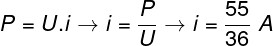Then, as the headlights are connected in series to the same fuse, we must consider that twice the current will flow through this fuse, so: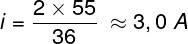Based on the result and the table, the fuse indicated for the function is orange, so the correct alternative is the letter C .

## Solved exercises on electrodynamics

Question 1 — Determine the magnitude of the electric current in a wire whose cross section is crossed by 5 x 10 20 electrons every second. Data: e = 1,6.10 -19 C.

a) 60 A

b) 80 A

c) 30 A

d) 50 A

Resolution:

Let’s use the electric current formula that takes into account the quantization of the electric charge .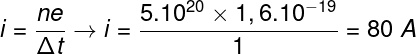Based on our result, the correct alternative is letter B.

Question 2 — Determine the magnitude of the electric current flowing through a conducting wire whose electrical resistance is equal to 0.005 Ω and which is subjected to a potential difference of 10V.

a) 2 mA

b) 2 kA

c) 50 mA

d) 0.5 kA

Resolution:

To resolve the issue, it is necessary to use Ohm’s 1st law.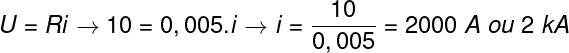Based on the calculation, the correct answer is letter B.

Question 3 — Determine the electrical power dissipated as heat in a wire of electrical resistance equal to 0.025 Ω, carrying an electric current of 20.0 A.

a) 10 W

b) 0.25 W

c) 0.5 W

d) 5.0 W

Resolution :

To solve the exercise, we must calculate the electrical power dissipated: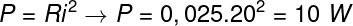Based on the calculation performed, the correct alternative is the letter A .